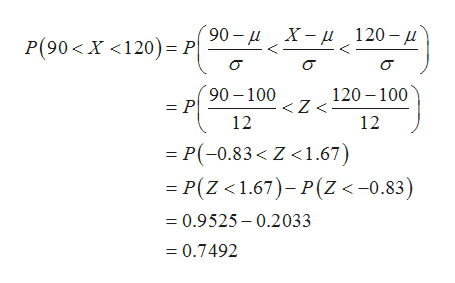# The monthly utility bills in a city are normally distributed with a mean of \$100 and a standard deviation of \$12. Find the probability that a randomly selected utility bill will be between \$90 and \$120.Distribution type: ________________   Probability: ________________

Question
414 views

The monthly utility bills in a city are normally distributed with a mean of \$100 and a standard deviation of \$12. Find the probability that a randomly selected utility bill will be between \$90 and \$120.

Distribution type: ________________   Probability: ________________

check_circle

Step 1

Let x is random variable.

Step 2

Given:

Distribution type: Normal distribution

Mean=\$100

Standard deviation=\$12

Step 3

The probability that a randomly selected utility bill will...help_outlineImage Transcriptionclose90- д Х— д 120- д Р(90 <х <120)— P о о о 120 100

### Want to see the full answer?

See Solution

#### Want to see this answer and more?

Solutions are written by subject experts who are available 24/7. Questions are typically answered within 1 hour.*

See Solution
*Response times may vary by subject and question.
Tagged in

### Statistics